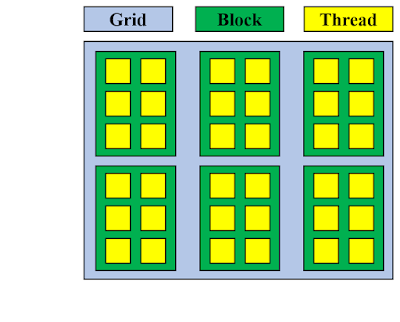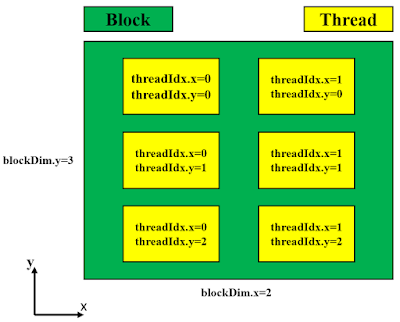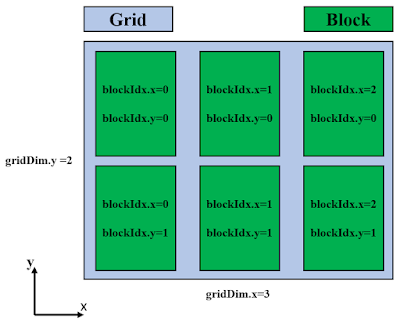To understand and implement CUDA codes in practice, the very first step is to understand the allocation of threads on GPU. Fundamentally, threads are basic units on GPU where computation can happen in a parallel way. To the first level, threads are grouped into blocks, either in a 1D, 2D or 3D manner, where each thread within a certain block has its own “coordinate” - a unique index for pinning down the location of a certain thread in the block. At the second level, blocks are further grouped into grid, in a similar way as how threads are grouped into block. Here we use three diagrams showing the basic idea of such a division manner for GPU computation units and how we really locate a thread in a block and a block in a grid.1 2 3 4 5 6 7 8 9 10 11 12 13 14 15 16 17 18 19 20 21 22 23 24 25 26 27 28 29 30 31 32 33 34 35 36 37 38 39 40 41 42 #include __global__ void saxpy(int n, float a, float *x, float *y) { int i = blockIdx.x*blockDim.x + threadIdx.x; if (i < n) y[i] = a*x[i] + y[i]; } int main(void) { int N = 1<<20; float *x, *y, *d_x, *d_y; x = (float*)malloc(N*sizeof(float)); y = (float*)malloc(N*sizeof(float)); cudaMalloc(&d_x, N*sizeof(float)); cudaMalloc(&d_y, N*sizeof(float)); for (int i = 0; i < N; i++) { x[i] = 1.0f; y[i] = 2.0f; } cudaMemcpy(d_x, x, N*sizeof(float), cudaMemcpyHostToDevice); cudaMemcpy(d_y, y, N*sizeof(float), cudaMemcpyHostToDevice); // Perform SAXPY on 1M elements saxpy<<<(N+255)/256, 256>>>(N, 2.0f, d_x, d_y); cudaMemcpy(y, d_y, N*sizeof(float), cudaMemcpyDeviceToHost); float maxError = 0.0f; for (int i = 0; i < N; i++) maxError = max(maxError, abs(y[i]-4.0f)); printf("Max error: %f\n", maxError); cudaFree(d_x); cudaFree(d_y); free(x); free(y); } 

One can save the codes as a file with extension ‘.cu’ and compile the codes using ‘nvcc’ compiler provided by NVIDIA (Click Me!), just like usually what we do for compiling normal C codes, e. g. by executing ‘nvcc -o first_cuda_demo first_cuda_demo.cu’. Here, we won’t go into further details about the code (refer to Ref.  for more introduction), and instead we will give here an abstract skeleton of the codes to get a bird view.Also, one can define all the CUDA relevant executions as a dedicated and callable routine. Then we can call the CUDA routine from normal C, C++, Fortran or whatever relevant codes. For example, we can write the codes above in a manner as shown below.
First, the caller C codes,

  1 2 3 4 5 6 7 8 9 10 11 12 13 14 15 16 17 18 19 20 21 22 23 24 25 26 27 28 29 #include #include #include #include extern void cuda_kernel(float *x, float *y, int N); int main(int argc, char *argv[]) { int N = 1<<20; float *x, *y; x = (float*)malloc(N*sizeof(float)); y = (float*)malloc(N*sizeof(float)); for (int i = 0; i < N; i++) { x[i] = 1.0f; y[i] = 2.0f; } cuda_kernel(&x, &y, N); float maxError = 0.0f; for (int i = 0; i < N; i++) { maxError = max(maxError, abs(y[i]-4.0f)); } printf("Max error: %f\n", maxError); return 0; } 

Saving the codes above as, e. g. ‘caller.c’.
Then the callee CUDA codes,

  1 2 3 4 5 6 7 8 9 10 11 12 13 14 15 16 17 18 19 20 21 22 23 24 25 26 27 #include __global__ void saxpy(int n, float a, float *x, float *y) { int i = blockIdx.x*blockDim.x + threadIdx.x; if (i < n) y[i] = a*x[i] + y[i]; } int cuda_kernel(float *x, float *y, int N) { float *d_x, *d_y; cudaMalloc(&d_x, N*sizeof(float)); cudaMalloc(&d_y, N*sizeof(float)); cudaMemcpy(d_x, x, N*sizeof(float), cudaMemcpyHostToDevice); cudaMemcpy(d_y, y, N*sizeof(float), cudaMemcpyHostToDevice); // Perform SAXPY on 1M elements saxpy<<<(N+255)/256, 256>>>(N, 2.0f, d_x, d_y); cudaMemcpy(y, d_y, N*sizeof(float), cudaMemcpyDeviceToHost); cudaFree(d_x); cudaFree(d_y); } 

Saving the callee CUDA codes above as, e. g., ‘callee.cu’.
Then use the Makefile provided below to compile the executable,

  1 2 3 4 5 6 7 8 9 10 11 12 13 14 CC=gcc NCC=nvcc OBJ=caller.o CUDA_OBJ=callee.o call_cuda: $(OBJ)$(CUDA_OBJ) $(CC) -o$@ $^$(OBJ): %.o: %.c $(CC) -c$< $(CUDA_OBJ): %.o: %.cu$(NCC) -c \$< 

Basically, during compiling, we can treat the CUDA codes just as normal C codes. Also, as usual, when library is used in the CUDA codes, we need to link to the libraries as we do for normal C codes (or whatever relevant codes, e. g., Fortran).
In the following blog, I will note down a slightly more complicated case - matrix multiplication with CUDA.

References